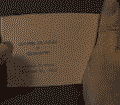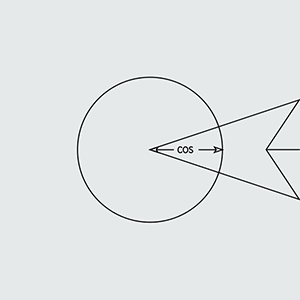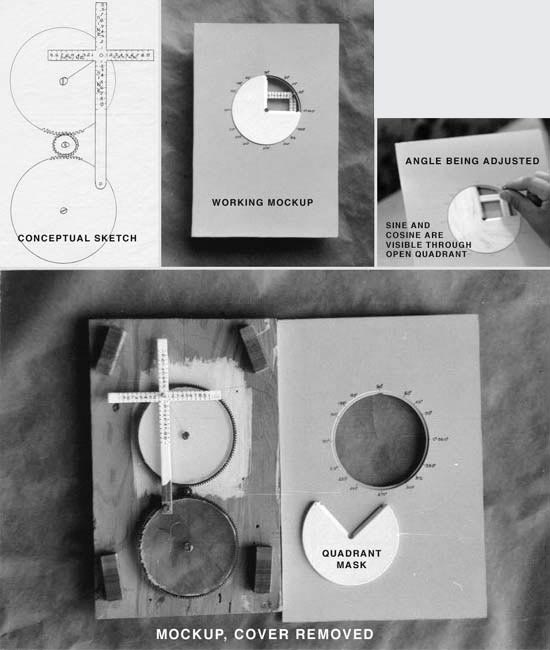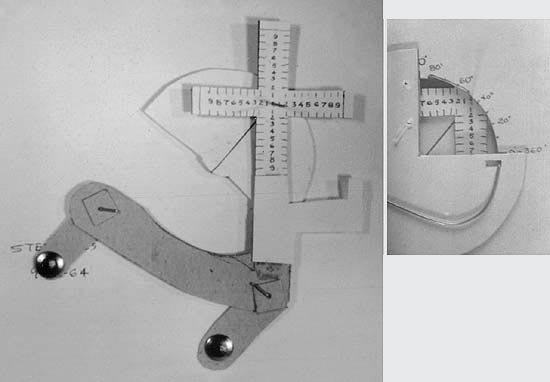# Trig Teaching Aid

Trigonometry is much easier to learn graphically.  Each of the six trig functions is represented in the diagram of an arm sweeping around a circle.Imagine a 2-inch diameter circle.  As an angle sweeps around the circle, all six trig functions can be seen increasing or decreasing.As the angle increases, the trig functions, change position (and therefore length) staying in relationship to the radius of one unit (1 inch, in this example) which corresponds to its numeral value listed for that trigonometric function.          This “trig machine” would best be used as a teaching aid.  Accuracy equal to a slide rule should be possible in a small model for student use.

The values of the tangent, cotangent, secant, and cosecant range from zero to infinity, however the machine achieves a false infinity marking so that the machine can be made a practical size.

As the angle indicator crosses the points marked 90, 180, 270, and 360 degrees the quadrant mask should snap from one quadrant to the next, exposing only the quadrant containing the angle pointed to by the indicator.Cardboard and thumbtack mockup.